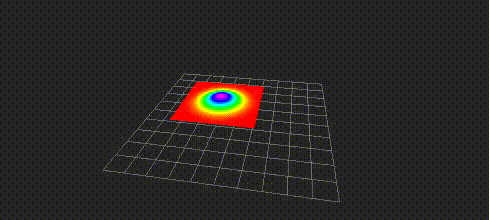# PoseWithCovarianceStampedToGaussianPointCloud¶Visualize geometry_msgs/PoseWithCovarianceStamped as gaussian pointcloud. Pointcloud is computed within a region of 3 sigma.

## Subscribing Topics¶

• ~input (geometry_msgs/PoseWithCovarianceStamped)

Input pose

## Publishing Topics¶

• ~output (sensor_msgs/PointCloud2)

Output pointcloud

## Parameters¶

• ~cut_plane (String, default: xy)

You can choose a plane to compute gaussian distribution from xy, yz, zx, flipped_xy, flipped_yz or flipped_zx.

• ~sampling_num (Int, default: 100)

The number of sampling for each axis. The number of points will square of ~sampling_num.

• ~normalize_method (String, default: normalize_area)

• ~normalize_value (Float, default: 1.0)

You can choose normalize_area or normalize_height as a method to normalize gaussian distribution.

If you choose normalize_area, area of gaussian distribution will be ~normalize_value.

If you choose normalize_height, the maximum height of gaussian distribution will be ~normalize_value.

## Sample¶

roslaunch jsk_pcl_ros_utils sample_pose_with_covariance_stamped_to_gaussian_pointcloud.launch